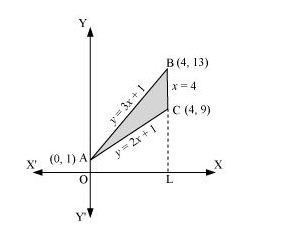# Using integration find the area of the triangular region whose sides have the equations

Question:

Using integration find the area of the triangular region whose sides have the equations $y=2 x+1, y=3 x+1$ and $x=4$.

Solution:

The equations of sides of the triangle are $y=2 x+1, y=3 x+1$, and $x=4$.

On solving these equations, we obtain the vertices of triangle as $A(0,1), B(4,13)$, and $C(4,9)$.It can be observed that,

Area (ΔACB) = Area (OLBAO) –Area (OLCAO)

$=\int_{0}^{4}(3 x+1) d x-\int_{0}^{4}(2 x+1) d x$

$=\left[\frac{3 x^{2}}{2}+x\right]_{0}^{4}-\left[\frac{2 x^{2}}{2}+x\right]_{0}^{4}$

$=(24+4)-(16+4)$

$=28-20$

$=8$ units